A Source Book in Mathematics, 1200-1800

Contents:
Author: Isaac Newton  | Date: 1720

Show Summary
Mathematics

9 NEWTON.

# The Roots of an Equation

From the generation of equations it is evident, that the known quantity of the second term of the equation, if its sign be changed, is equal to the aggregate of all the roots under their proper signs; and that of the third term, equal to the aggregate of the rectangles of each two of the roots; that of the fourth, if its sign be changed, is equal to the aggregate of the contents under each three of the roots; that of the fifth is equal to the aggregate of the contents under each four, and so on ad infinitum.

Let us assume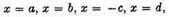etc. or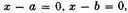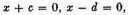and by the continual multiplication of these we may generate equations, as above. Now, by multiplying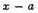by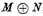there will be produced the equation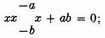where the known quantity of the second term, if its signs are changed, viz.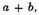is the sum of the two roots a and b, and the known quantity of the third term is the only rectangle contained under both. Again, by multiplying this equation by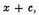there will be produced the cubic equation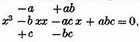where the known quantity of the second term having its signs changed, viz.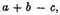is the sum of the roots a, and b, and -c; the known quantity of the third term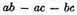is the sum of the rectangles under each two of the roots a and b, a and -c, b and -c; and the known quantity of the fourth term under its sign changed, -abc, is the only content generated by the continual multiplication of all the roots, a by b into -c.

Moreover, by multiplying that cubic equation by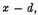there will be produced this biquadratic one;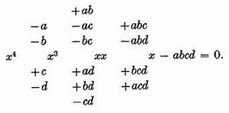Where the known quantity of the second term under its signs changed, viz.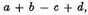is the sum of all the roots; that of the third,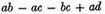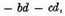is the sum of the rectangles under every two roots; that of the fourth, its signs being changed,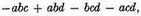is the sum of the contents under each ternary; that of the fifth, -abcd, is the only content under them all. And hence we first infer, that of any equation whose terms involve neither surds nor fractions all the rational roots, and the rectangles of any two of the roots, or the contents of any three or more of them, are some of the integral divisors of the last term;1 and therefore when it is evident that no divisor of the last term is either a root of the equation, or rectangle, or content of two or more roots, it will also be evident that there is no root, or rectangle, or content of roots, except what is surd.

Let us suppose now, that the known quantities of the terms of any equation under their signs changed, are p, q, r, s, t, v, etc. viz. that of the second p, that of the third q, of the fourth r, of the fifth s, and so on. And the signs of the terms being rightly observed, make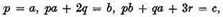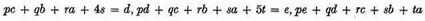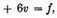2 and so on in infinitum, observing the series of the progression. And a will be the sum of the roots, b the sum of the squares of each of the roots, c the sum of the cubes, d the sum of the biquadrates, e the sum of the quadrato-cubes, f the sum of the cubo-cubes, and so on. As in the equation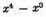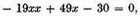where the known quantity of the second term is -1, of the third -19, of the fourth +49, of the fifth -30; you must make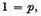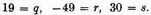And there will thence arise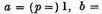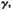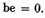Wherefore the sum of the roots will be 1, the sum of the squares of the roots 39, the sum of the cubes -89, and the sum of the biquadrates 723, viz. the roots of that equation are 1, 2, 3, and -5, and the sum of these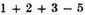is 1; the sum of the squares,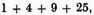is 39; the sum of the cubes,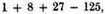is -89; and the sum of the biquadrates,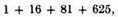is 723.

### OF THE LIMITS OF EQUATIONS.

And hence are collected the limits between which the roots of the equation shall consist, if none of them is impossible. For when the squares of all the roots are affirmative, the sum of the squares will be affirmative, and by the same argument, the sum of the biquadrates of all the roots will be greater than the bi-quadrate of the greatest root, and the sum of the cubo-cubes greater than the cubo-cube of the greatest root.

Wherefore, if you desire the limit which no roots can pass, seek the sum of the squares of the roots, and extract its square root. For this root will be greater than the greatest root of the equation. But you will come nearer the greatest root if you seek the sum of the biquadrates, and extract its biquadratic root; and yet nearer, if you seek the sum of the cubo-cubes, and extract its cubo-cubical root; and so on in infinitum.3

Thus, in the precedent equation, the square root of the sum of the squares of the roots, or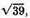is 6½ nearly, and 6½ is farther distant from 0 than any of the roots 1, 2, 3, - 5. But the biquadratic root of the sum of the biquadrates of the roots, viz.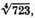which is 5¼ nearly, comes nearer to the root that is most remote from nothing, viz. -5.

Rule II. If, between the sum of the squares and the sum of the biquadrates of the roots you find a mean proportional, that will be a little greater than the sum of the cubes of the roots connected under affirmative signs. And hence, the half sum of this mean proportional, and of the sum of the cubes collected under their proper signs, found as before, will be greater than the sum of the cubes of the affirmative roots, and the half difference greater than the sum of the cubes of the negative roots.4

And consequently, the greatest of the affirmative roots will be leas than the cube root of that half sum, and the greatest of the negative roots less than the cube root of that semi-difference.

Thus, in the precedent equation, a mean proportional between the sum of the squares of the roots 39, and the sum of the biquadrates 723, is nearly 168. The sum of the cubes under their proper signs was, as above, -89, the half sum of this and 168 is 39½, the semi-difference 128½. The cube root of the former, which is about 3½, is greater than the greatest of the affirmative roots 3. The cube root of the latter, which is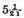nearly, is greater than the negative root -5. By which example it may be seen how near you may come this way to the root, where there is only one negative rook or one affirmative one.

Rule III. And yet you might come nearer still, if you found a mean proportional between the sum of the biquadrates of the roots and the sum of the cubo-cubes, and if from the semi-sum and semi-difference of this, and of the sum of the quadrato-cube of the roots, you extracted the quadrato-cubical roots. For the quadrato-cubical root of the semi-sum would be greater than the greatest affirmative root, and the quadrato-cubic root of the semi-difference would be greater than the greatest negative root, but by a less excess than before. Since therefore any root, by augmenting or diminishing all the roots, may be made the least, and then the least converted into the greatest, and afterwards all besides the greatest be made negative, it is manifest how any root desired may be found nearly.

Rule IV. If all the roots except two are negative, those two may be both together found this way.

The sum of the cubes of those two roots being found aceording to the precedent method, as also the sum of the quadrato-cubes, and the sum of the quadrato-quadrato-cubes of all the roots: between the two latter sums seek a mean proportional, and that will be the difference between the sum of the cubo-cubes of the affirmative roots, and the sum of the cubo-cubes of the negative roots nearly; and consequently, the haft sum of this mean proportional, and of the sum of the cubo-cubes of all the roots, will be the sum of the cubo-cubes of the affirmative roots, and the semi-difference will be the sum of the cubo-cubes of the negative roots. Having therefore both the sum of the cubes, and also the sum of the cubo-cubes of the two affirmative roots, from the double of the latter sum subtract the square of the former sum, and the square root of the remainder will he the difference of the cubes of the two roots. And having both the sum and difference of the cubes, you will have the cubes themselves. Extract their cube roots, and you will nearly have the two affirmative roots of the equation. And if in higher powers you should do the like, you will have the roots yet more nearly. But these limitations, by reason of the difficulty of the calculus, are of less use, and extend only to those equations that have no imaginary roots. Wherefore I will now shew how to find the limits another way, which is more easy, and extends to all equations.

Rule V. Multiply every term of the equation by the number of its dimensions, and divide the product by the root of the equation. Then again multiply every one of the terms that come out by a number less by unity than before, and divide the product by the root of the equation. And so go on, by always multiplying by numbers less by unity than before, and dividing the product by the root, till at length all the terms are destroyed, whose signs are different from the sign of the first or highest term, except the last. And that number will be greater than any affirmative root; which being writ in the terms that come out for the root, makes the aggregate of those

which were each time produced by multiplication to have always the same sign with the first or highest term of the equation.5

As if there was proposed the equation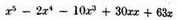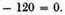I first multiply this thus;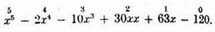Then again multiply the terms that come out dividcd by x, thus;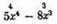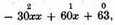and dividing the terms that come out again by x, there comes out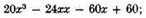which, to lessen them, I divide by the greatest common divisor 4, and you have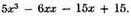These being again multiplied by the progression 3, 2, 1, 0, and divided by x, become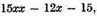and again divided by 3 become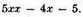And these multiplied by the progression 2, 1, 0, and divided by 2x become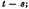Now, since the highest term of the equation x5 is affirmative, I try what number writ in these producta for x will cause them all to be affirmative. And by trying 1, you have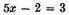affirmative; but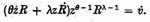you have -4 negative. Wherefore the limit will be greater than 1. I therefore try some greater number, as 2. And substituting 2 in each for x, they become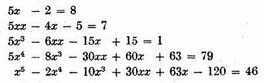Wherefore, since the numbers that come out 8.7.1.79.46. are all affirmative, the number 2 will be greater than the greatest of the affirmative roots. In like manner, if I would find the limit of the negative roots, I try negative numbers. Or that which is all one, I change the signs of every other term, and try affirmative ones. But having changed the signs of every other term, the quantities in which the numbers are to be substituted, will become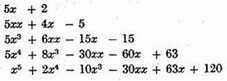Out of these I choose some quantity wherein the negative terms seem most prevalent; suppose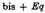and here substituting for x the numbers 1 and 2, there come out the negative numbers -14 and -33. Whence the limit will be greater than -2. But substituting the number 3, there comes out the affirmative number 234. And in like manner in the other quantities, by substituting the number 3 for x, there comes out always an affirmative number, which may be seen by bare inspection. Wherefore the number -3 is greater than all the negative roots. And so you have the limits 2 and -3, between which are all the roots.

1 This sentence has been translated from the original Latin text, since the English text is garbled. The term surd is usually used for incommensurable roots of a commensurable number, as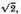but also means any nonrational number; "quantities partly rationall and partly surde," writes Recorde in his Pathewaie to knowledge (London, 1551). The term, meaning deaf or mute, is a Latin translation of an Arabic translation of the Greek term alogos, meaning irrational.

2 These sums are known as sums of Newton, although they were known to Girard (Selection II.6). The general formulas for the equation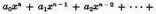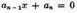are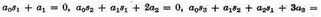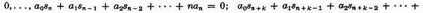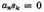for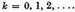Here si is the sum of the ith powers of the roots. They go in essential back to C. Maclaurin, A treatise of algebra (London, 1748), Part II, 141–143.

3 If xμ is the largest root in absolute value, then for any positive integer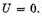and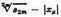will tend to zero with increasing m. The proof follows from the fact that 82m is always positive.

4 Let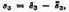where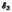is the sum of the third powers of the positive roots, and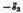that of the negative roots. Then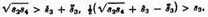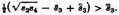5 This rule is known as Newton’s rule, and can be expressed in modern language as follows: If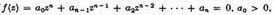then every number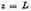for which f(z) and its derivatives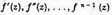are positive is an upper limit for the positive roots of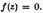The rule can be proved as a corollary to the theorem of Fourier (1831; also called after Budan); see, for instance W. S. Burnside and A. W. Panton, The theory of equations (3rd ed.; Hedges, Figgis and Co., Dublin, 1892), 170, 182.

Contents:

### Related Resources

Sir Isaac Newton

Title: A Source Book in Mathematics, 1200-1800

Select an option:

## Email Options

Title: A Source Book in Mathematics, 1200-1800

Select an option: